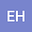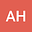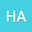A capable numerical meshless scheme for solving distributed order time-fractional reaction-diffusion equation
•••Distributed order fractional differential equations are efficient in describing physical phenomena because of the differential order distribution. In this paper, the distributed order time-fractional reaction-diffusion equation is considered in the sense of Caputo fractional derivative. A hybrid method is developed based on the Moving Kriging (MK) interpolation and finite difference method for solving this distributed order equation. First, the distributed integral is discretized by the $M$-point Gauss Legendre quadrature rule. Then, the $L2-1_{\sigma}$ method is applied to approximate the solution of the fractional derivative discretization. Also, the unconditionally stability and rate of convergence $O(\tau^{2})$ of the time-discrete technique are illustrated. Furthermore, the MK interpolation is applied in the space variables discretization. Finally, some examples are presented to indicate the efficiency of this method and endorsement the theoretical results.